IF YOU CAN SEE THIS go to /sfa/Nuprl/Shared/Xindentation_hack_doc.html

### Types: Ontic, Semantic, and Intensional

A type in computational type theory is essentially a class of values, such a class being the domain of some function. Further, since we adopt a constructive account of functions and classes, the type must be a method for notating such values, and in such a way that whatever computations one might apply to those values can be expressed as a computation on those notations.

Because of the intimate connection between values and notations in constructive mathematical notation, there are two manners, or "moods", of discourse involving types (ontic and semantic). There are two corresponding notions of "type", (ontic and semantic), plus a third notion of type, "intensional type", which is exploited in the formulation of Nuprl's logic (see Intensional Types). The word "type" is used for any of these, as well as being used to mean an expression referring to a type in any of these senses (see Type Expressions).

Ontic Types and Ontic Mood.

The ontic "mood" is that of a priori discourse about values; it is the one most like informal, pre-theoretical discourse. Thus, one talks about numbers, functions, pairs, etc. In this mood, a type is a class of values, and a type expression stands for such a class.
In the ontic mood, "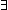x: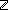. 0 = x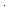x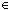" might be glossed as simply "Some integer multiplied by itself is 0". More simply, "1+2 = 3" might be glossed as "the sum of integers 1 and 2 is 3".
It is the formalization of the ontic mood that the logic of computational type theory aims at, but to do this carefully requires us to engage in a form of semantic discourse.

Semantic Types and Semantic Mood.

The semantic mood is that of discourse about expressions and reference. For example, "1+2 = 3" might be glossed as "the expressions 1+2 and 3 denote the same value of type"; or we might say "the expressions 1+2 and 3 satisfy the symmetric-transitive relation on terms stipulated as the meaning of type expression" if we want to emphasize the general form of the semantics of types used in computational type theory.

The general semantic form we adopt for types is to associate with each type expression a so-called partial equivalence relation, i.e. a symmetric transitive relation, on expressions; this relation is called the "equality" of the type. The semantics of computational type theory requires a notion of "expression" whose syntax and execution semantics is independent of the definition of types. Restrictions on candidates for an equality relation include that any expression satisfying the relation is closed, i.e. has no free variables, and that if tT and t' is Kleene-equal to t, then t = t'T. Two expressions are Kleene-equal just when, if either one yields a value upon execution, the other yields the same value. See Computation. This semantic form is directly exploited in Comprehension Subtypes and Quotient Types.

As a practical matter, Nuprl further requires that rewriting any subexpression of an expression, of whatever type, by a step of computation must result in an "equal" term of that type. It turns out that this constraint will follow from a few painless restrictions on the computation and type definition, which we do not further discuss here; this result is due to Doug Howe (see also "Squiggle"). It is also required that change of bound variables results in an equal expression; while this must be considered at type definition time, it does not complicate type definition, and you'd have to go out of your way when designing the type system to violate it.

Intensional Types are partial equivalence classes of Type Expressions.

Ontic vs Semantic.

So, in the ontic mood, we may truly say that the boolean type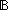has exactly two members, whereas in the semantic mood, this would be false, since one can always make a larger expression that stands for a given value (if tT then t = (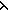x.x)(tT, for example).
In the semantic mood, "0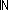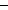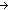" is false, whereas in the ontic mood, "0" is assigned no sense at all. See Equality.

One tends to use both moods since in order to be able to talk intuitionistically about a class of values, one must adopt a notation for those values that is computationally adequate.

Standard examples are natural numbers and functions.
A notation for natural numbers counts as constructively adequate just when one can effectively determine from any notation for a number whether that number is zero, and if it is not, one can effectively find a notation for its predecessor.
Let us suppose we have such a method for notating numbers, and call it.
A notation for a function, say from naturals to naturals, using the notational method, counts as constructively adequate just when, from any expression none can effectively find an expression offor the value of that function on n.

(March 2001 - sfa )